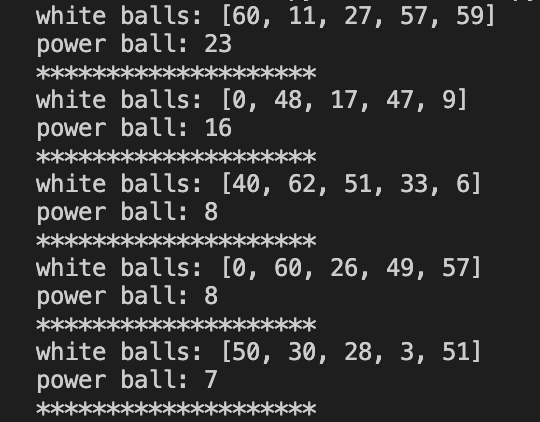## Win the Lottery With Python

### by IoFAdmin at Oct 10, 2021

#### python | programming#### We're Going To Be Rich!

Rich with Python knowledge that is... If you do win millions of dollars with this mini project, please send me some cash too! As Mr. T would say when I was a kid, that's enough jibba jabba so let's get started.#### How Powerball Works

In case you're not familar with the rules of Powerball, you can read them here.

#### Random Numbers

To simulate Powerball picks, we're going to need some random numbers. If you're new to programming and/or Python then you may not know much about generating random numbers. Our code will pick a number between MIN_NUMBER and MAX_NUMBER, once for each ball in our simulation. Computers can't actually generate totally random numbers but we're not launching rockets so our pseudo random numbers are good enough.

#### Let's Get "Classy"

We're going to represent our Professional Powerball Picker (is that really a job?) with a Python class that we'll call Powerball. Not very imaginative naming but I digress. We'll instantiate this class and then simulate NUM_OF_DRAWS draws.

#### Our Dependencies

Our Powerball class is mostly self-contained but we'll need to use Python's built-in random module. Check out the official docs for more on random.

#### Bring On the Code

I'll show you all of the code and then we'll break it down in easy to understand chunks. Kinda like knowledge nuggets... without the dipping sauce.

```from random import shuffle, randint

class Powerball:
NUM_BALLS = 5
MAX_BALL = 69
MAX_POWERBALL = 26
NUM_OF_DRAWS = 5

def __init__(self) -> None:
self.balls = list()
self.balls_selected = list()
self.powerball = None

def setup(self) -> None:
self.balls = [i for i in range(self.MAX_BALL)]
shuffle(self.balls)

self.selectBalls()
self.selectPowerball()

def selectBalls(self) -> None:
self.balls_selected = [self.balls.pop() for i in range(self.NUM_BALLS)]

def selectPowerball(self) -> None:
self.powerball = randint(1, self.MAX_POWERBALL)

def showResults(self) -> None:
print(f'white balls: {self.balls_selected}')
print(f'power ball: {self.powerball}')
print('*'*20)

if __name__ == '__main__':
powerball = Powerball()

for x in range(powerball.NUM_OF_DRAWS):
powerball.setup()
powerball.showResults()```

Let's start at the beginning... because the end would be silly.

```if __name__ == '__main__':
powerball = Powerball()

for x in range(powerball.NUM_OF_DRAWS):
powerball.setup()
powerball.showResults()```

The if __name__=='__main__': part protects users from accidentally running the code if they import it in. If you want more information on that StackOverflow has a great post but you can probably ignore it for now.

Then we create a new instance of the Powerball class and save it to the powerball variable. Finally, we loop NUM_OF_DRAWS times and make our picks.

```class Powerball:
NUM_BALLS = 5
MAX_BALL = 69
MAX_POWERBALL = 26
NUM_OF_DRAWS = 5```

We define our Powerball class and then define four variables which are known as member variables in object oriented lingo. We're going to use them as constants even though Python doesn't really support constants like some other programming languages do.

```def __init__(self) -> None:
self.balls = list()
self.balls_selected = list()
self.powerball = None```

With our init method, we're telling our users that we're not going to return anything (-> None) and we define two lists and a None type variable. We'll set the value of these variables shortly.

```def setup(self) -> None:
self.balls = [i for i in range(self.MAX_BALL)]
shuffle(self.balls)

self.selectBalls()
self.selectPowerball()```

Here's our setup method which also returns nothing. The first line of our method is using list comprehension to create integers from 1 to MAX_BALL and saving them into our self.balls variable. Then we're using shuffle to randomly shuffle the order of the integers of our list. Finally we call two methods to actually select our numbers.

```def selectBalls(self) -> None:
self.balls_selected = [self.balls.pop() for i in range(self.NUM_BALLS)]```

Our aptly named selectBalls method lives up to its namesake: it selects our balls. The for i in range(self.NUM_BALLS) code is taking the first NUM_BALLS balls and self.balls.pop() is removing them from the list. Then finally we're saving those selected balls to the self.balls_selected variable. So to sum it up, at the end of our method we'll have 5 integers in our list. Note that we're not randomizing anything in this method because we already shuffled the list in the setup method.

```def selectPowerball(self) -> None:
self.powerball = randint(1, self.MAX_POWERBALL)```

Our selectPowerball method also returns nothing. We use the randint function to randomly select a number between 1 and MAX_POWERBALL and then we save it to the self.powerball variable.

```def showResults(self) -> None:
print(f'white balls: {self.balls_selected}')
print(f'power ball: {self.powerball}')
print('*'*20)```

The showResults method is very simple: just show results. First we print the white balls and then we print our powerball. Finally, we print a divider made of asterisks.